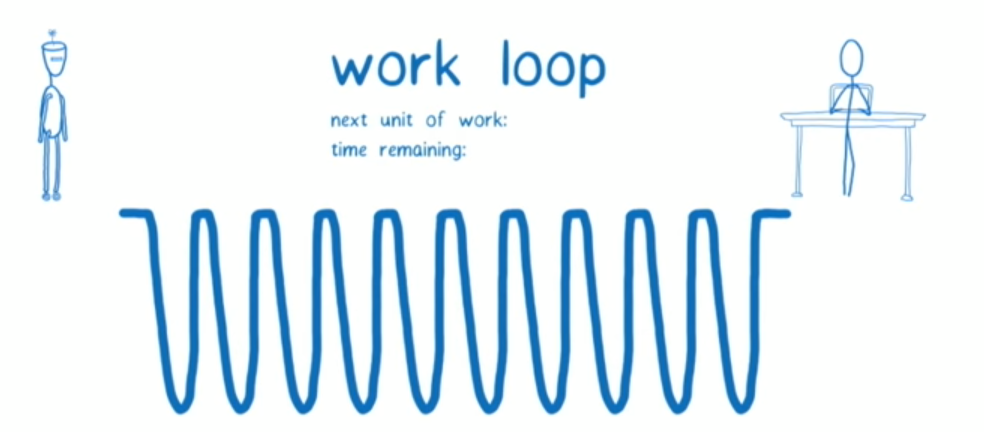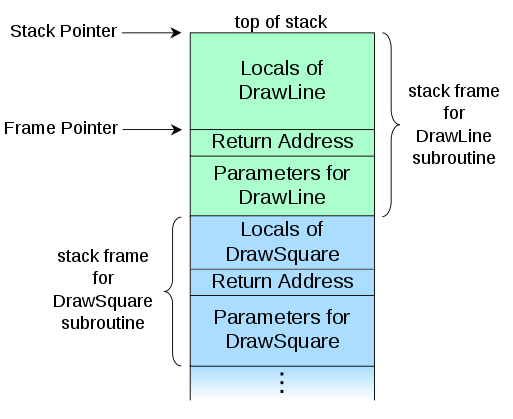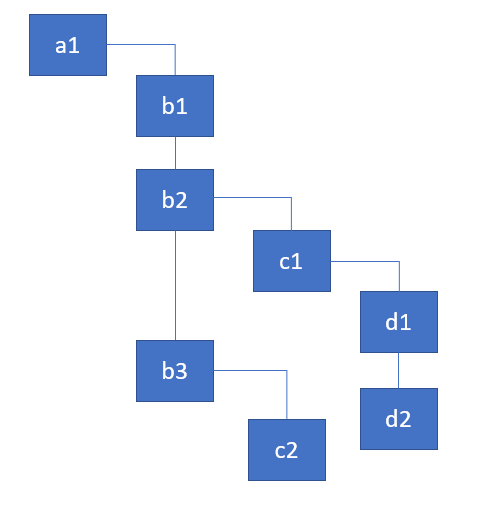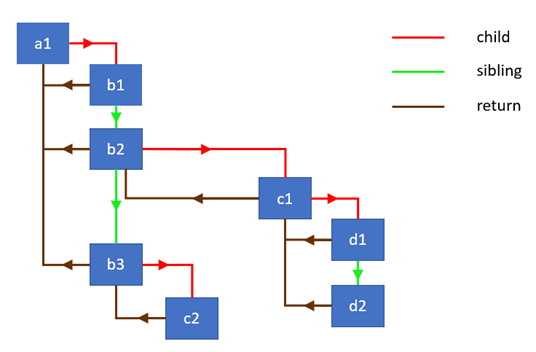React如何以及为何在Fiber架构中使用链表结构来遍历组件树### Setting the background（背景）

Fiber 架构主要有两大阶段：reconciliation / render （调协）以及 commit （提交）。在源码中，reconciliation 阶段大多称为 “render phase”。这个阶段，React 遍历组件树并完成下边几个事：

• 更新状态、属性
• 调用生命周期函数
• 获取组件的子元素
• 和之前的子元素进行比较
• 计算出需要需要做怎样的DOM操作

When dealing with UIs, the problem is that if too much work is executed all at once, it can cause animations to drop frames…

Newer browsers (and React Native) implement APIs that help address this exact problem…

Andrew 提到的这个 API 就是 requestIdleCallback，它能将一个函数加入队列，并在浏览器空闲的时候来执行之。

``````requestIdleCallback((deadline)=>{
});``````

requestIdleCallback is actually a bit too restrictive and is not executed often enough to implement smooth UI rendering, so React team had to implement their own version.

``````requestIdleCallback((deadline) => {
// while we have time, perform work for a part of the components tree
nextComponent = performWork(nextComponent);
}
});``````

in order to use those APIs, you need a way to break rendering work into incremental units

If you rely only on the [built-in] call stack, it will keep doing work until the stack is empty…Wouldn’t it be great if we could interrupt the call stack at will and manipulate stack frames manually? That’s the purpose of React Fiber. Fiber is re-implementation of the stack, specialized for React components. You can think of a single fiber as a virtual stack frame.

In computer science, a call stack is a stack data structure that stores information about the active subroutines of a computer program… the main reason for having call stack is to keep track of the point to which each active subroutine should return control when it finishes executing… A call stack is composed of stack frames… Each stack frame corresponds to a call to a subroutine which has not yet terminated with a return. For example, if a subroutine named DrawLine is currently running, having been called by a subroutine DrawSquare, the top part of the call stack might be laid out like in the adjacent picture.### 为什么 stack 和 React 有关

By default, when recursing on the children of a DOM node, React just iterates over both lists of children at the same time and generates a mutation whenever there’s a difference.render 方法返回的是一些对象的数组，将它们看作组件实例即可：

``````const a1 = {name: 'a1'};
const b1 = {name: 'b1'};
const b2 = {name: 'b2'};
const b3 = {name: 'b3'};
const c1 = {name: 'c1'};
const c2 = {name: 'c2'};
const d1 = {name: 'd1'};
const d2 = {name: 'd2'};

a1.render = () => [b1, b2, b3];
b1.render = () => [];
b2.render = () => [c1];
b3.render = () => [c2];
c1.render = () => [d1, d2];
c2.render = () => [];
d1.render = () => [];
d2.render = () => [];``````

React 需要迭代这个树结构，并为每个组件执行任务。为简化起见，要做的任务是打印出当前组件的名字并获取子元素。

### Recursive traversal （递归遍历）

``````walk(a1);

function walk(instance) {
doWork(instance);
const children = instance.render();
children.forEach(walk);
}

function doWork(o) {
console.log(o.name);
}``````

``a1, b1, b2, c1, d1, d2, b3, c2``

• child —— 指向第一个子节点
• sibling —— 指向第一个兄弟节点
• return —— 指向父节点``````class Node {
constructor(instance) {
this.instance = instance;
this.child = null;
this.sibling = null;
this.return = null;
}
}``````

``````function link(parent, elements) {
if (elements === null) elements = [];

parent.child = elements.reduceRight((previous, current) => {
const node = new Node(current);
node.return = parent;
node.sibling = previous;
return node;
}, null);

return parent.child;
}``````

``````const children = [{name: 'b1'}, {name: 'b2'}];
const parent = new Node({name: 'a1'});

// the following two statements are true
console.log(child.instance.name === 'b1');
console.log(child.sibling.instance === children);``````

``````function doWork(node) {
console.log(node.instance.name);
const children = node.instance.render();
}``````

``````function walk(o) {
let root = o;
let current = o;

while (true) {
// perform work for a node, retrieve & link the children
let child = doWork(current);

// if there's a child, set it as the current active node
if (child) {
current = child;
continue;
}

// if we've returned to the top, exit the function
if (current === root) {
return;
}

// keep going up until we find the sibling
while (!current.sibling) {

// if we've returned to the top, exit the function
if (!current.return || current.return === root) {
return;
}

// set the parent as the current active node
current = current.return;
}

// if found, set the sibling as the current active node
current = current.sibling;
}
}``````Fiber is re-implementation of the stack, specialized for React components. You can think of a single fiber as a virtual stack frame.

``````function walk(o) {
let root = o;
let current = o;

while (true) {
...

current = child;
...

current = current.return;
...

current = current.sibling;
}
}``````

### Work loop in React （React 中的任务循环）

React 里实现任务循环的代码在此：

``````function workLoop(isYieldy) {
if (!isYieldy) {
// Flush work without yielding
while (nextUnitOfWork !== null) {
nextUnitOfWork = performUnitOfWork(nextUnitOfWork);
}
} else {
// Flush asynchronous work until the deadline runs out of time.
while (nextUnitOfWork !== null && !shouldYield()) {
nextUnitOfWork = performUnitOfWork(nextUnitOfWork);
}
}
}``````